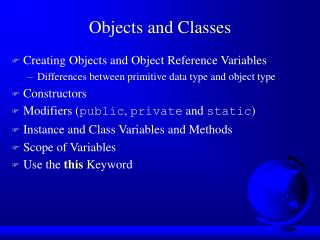# Objects and Classes - PowerPoint PPT PresentationDownload PresentationObjects and Classes

Download Presentation## Objects and Classes

- - - - - - - - - - - - - - - - - - - - - - - - - - - E N D - - - - - - - - - - - - - - - - - - - - - - - - - - -
##### Presentation Transcript

1. Objects and Classes • Creating Objects and Object Reference Variables • Differences between primitive data type and object type • Constructors • Modifiers (public, private and static) • Instance and Class Variables and Methods • Scope of Variables • Use the this Keyword

2. Class Concept

3. UML: Unified Modeling Language

4. Class Circle Declaration class Circle { double x0 = 0.0; double y0 = 0.0; double radius = 1.0; double findArea() { return radius*radius*3.14159; } }

5. Declaring Object Reference Variables ClassName objectName; Example: Circle myCircle;

6. Creating Objects objectName = new ClassName(); Example: myCircle = new Circle(); The object reference is assigned to the objectreference variable.

7. Declaring/Creating Objectsin a Single Step ClassName objectName = new ClassName(); Example: Circle myCircle = new Circle();

8. Test Class Circle public class TestCircle { public static main(String args[]) { double area1, area2; Circle myCircle1 = new Circle(); Circle myCircle2 = new Circle(); area1 = MyCircle1.findArea(); area2 = MyCircle2.findArea(); System.out.println (”S1=”+area1); System.out.println (”S2=”+area2); } }

9. Object reference The object reference is a handle to the location where the object resides in memory. When a primitive value is passed into a method, a copy of theprimitive is made. The copy is what is actually manipulated in the method. So, the value of the copy can be changed within the method, but the original value remains unchanged. When an object reference or array reference is passed into a method, a copy of thereference is actually manipulated by the method. So, the method can change the attributes of the object.

10. Accessing Objects • Referencing the object’s data: objectName.data Example:r=myCircle.radius; myCircle.radius=10.0; • Invoking the object’s method: objectName.method() Example:S = myCircle.findArea();

11. Constructors Constructors are a special kind of methods that are invoked to construct objects. Circle() { x0 = 0.0; y0 = 0.0; radius = 1.0; } Circle(double x, double y, double r) { x0 = x; y0 = y; radius = r; }

12. Constructors, cont. A constructor with no parameters is referred to as a default constructor. ·       Constructors must have the same name as the class itself. ·       Constructors do not have a return type—not even void. ·       Constructors are invoked using the new operator when an object is created. Constructors play the role of initializing objects.

13. Constructors and method for Class Circle public class Circle { ... Circle() // Default constructor { x0 = 0.0; y0 = 0.0; radius = 1.0; } Circle(double x, double y, double r) { x0 = x; y0 = y; radius = r; } findArea() { return radius*radius*3.14159; } }

14. Default Constructor • If a class does not define any constructor explicitly, a default constructor is defined implicitly. • If a class defines constructor implicitly, a default constructor does not exist unless it is defined explicitly.

15. Using Constructors • Objective: Demonstrate the role of constructors and use them to create objects. myCircle = new Circle(); myCircle = new Circle(2,.0,3.0,5.0);

16. Visibility Modifiers and Accessor Methods By default, the class, variable, or data can beaccessed by any class in the same package (will be explained later ). • public The class, data, or method is visible to any class in any package. • private The data or methods can be accessed only by the declaring class.

18. Instance Variables and Methods Instance variables belong to a specific instance.Instance methods are invoked by an instance of the class.

19. Class Variables, Constants, and Methods Classvariables are shared by all the instances of the class.Class methods are not tied to a specific object. Classconstants are final variables shared by all the instances of the class.

20. Class Variables, Constants, and Methods, cont. To declare class variables, constants, and methods, use the static modifier. private static int numberOfObjects=0; public final static double PI = 3.1415926;

21. Class Variables, Constants, and Methods, example. public class Circle { private static int numberOfObjects=0; public final static double PI = 3.1415926; Circle(double x, double y, double r) { x0=x; y0=y; radius=r; numberOfObjects++; } public static getNumberOfObjects() { return numberOfObjects; } … }

22. Class Variables, Constants, and Methods, cont.

23. Math is class with private constructor • Class Math contains only public static methods: Math.sin() Math.asin () Math.ceil() Math.round() Math.abs() Math.min() Math.pow() Math.exp() Math.sqrt() ...

24. Scope of Variables • The scope of instance and class variables is the entire class. They can be declared anywhere inside a class. • The scope of a local variable starts from its declaration and continues to the end of the block that contains the variable. A local variable must be declared before it can be used.

25. The Keyword this • Use this to refer to the current object. • Use this to invoke other constructors of the object. public void setRadius(double radius) { this.radius = radius; } public Circle() { this(0.0, 0.0, 1.0); }